# Module 1.5 - Control Actions

## The Controller

The controller plays an essential role in the control system. Of the four basic functions of a control system, (measurement, comparison, computation, and correction) comparison and computation are solely achieved by the controller. The correction is materialized by the final control element, but this is done according to the controller's calculation.

The control mechanism in the controller may be considered as consisting of two sections

• The Comparator
• The Controller

The purpose of the first is to compare the measured and the desired values of the controlled variable and then compute the difference between them as the error. If there is no error, i.e. the controlled variable is at the setpoint, then no action is taken.

If an error is detected, the second section of the controller operates to alter the setting of the final control element in such a way as to minimize the error in the least possible time with the minimum disturbance to the system. To achieve this objective, different actions could be taken by the controller and hence different signals are sent to the final control element.

The remainder of this section is concerned with the different control actions that the feedback controller could take.

## On-off or two-position action

This is the simplest and most commonly experienced type of control. A typical example is the thermostatically controlled domestic immersion heater. Depending on the temperature of the water in the tank, the power supply to the heater is either on or off. In The Virtual Control Laboratory and Case Study Section there is an experiment which shows on-off control of the temperature in a hot water tank.

Advantage of this type of control action is that it is inexpensive and extremely simple.

The disadvantage mainly lies in the oscillatory nature of the control, which makes it suitable only for those applications where it can be used alone and close control is not essential.

Note that it is impractical to use on-off control when trying to regulate a flowrate. It is necessary to have some sort of capacity available. This can be illustrated by means of an experiment which uses as a scenario someone taking a shower. Once again the experiment can be found in The Virtual Control Laboratory and Case Study Section

Thus the application of on/off control in industry is severely limited. In most industrial applications continuous control modes, such as those described below are widely adopted.

## Proportional Control Action

Proportional action is the simplest and most commonly encountered of all continuous control modes. In this type of action, the controller produces an output signal which is proportional to the error. Hence, the greater the magnitude of the error, the larger is the corrective action applied.

• ## Mathematical Description

Mathematically, proportional control could be expressed as: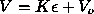Where
• V is the adjustment or signal for the adjustment from the controller.
•is the error.
•= S - L
• L is the measured value of the controlled variable.
• S is the setpoint.
• K is the proportional constant, named as the gain which shows the sensitivity of the control.
• Vo is the signal output when no error exists.

The gain is often replaced with another parameter, called the proportional band, PB. This quantity is defined as the error required to move the final control element over its whole range and is expressed as a percentage of the total range of the measured variable. What is the relationship between K and PB.

According to this definition we can see that the whole range of the final control element adjustment should be Vmin to Vmax.

At point Vmin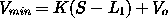At point Vmax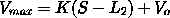The error required to move from Vmin to Vmax will be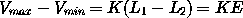Therefore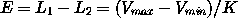Recall that the proportional band, PB, is defined as the error required to move the final control element over it's whole range expressed as a %. So for the controlled variable, L, with its total range Lmin to Lmax the definition for the proportional band is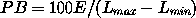or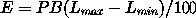Therefore we have the relationship between gain K and proportional band PB as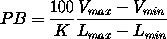With proportional band, the relationship between the adjustment and the error can be expressed as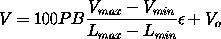It can be seen both from the expression above and by running the experiments in the Virtual Laboratory that the larger the gain K, or equivalently the smaller the proportional band PB, the higher the sensitivity of the controller's actuating signal to deviations will be.

• ## Dynamic Response

Now let's examine the dynamic response of the proportional control. Assume the process is at steady state and the level is at the setpoint. At time = 0, an increase in the inlet flowrate, regarded as a disturbance, enters into the process. If no control action is taken, i.e. the outlet flowrate is not altered, the level (controlled variable) will increase.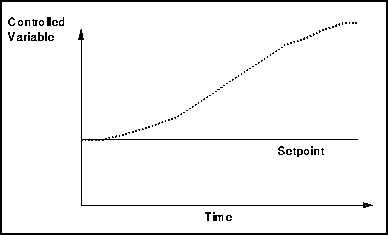With proportional control, the level is brought back and maintained in a certain range near the setpoint. The history curve could typically be like that shown below. Different responses are obtained depending on the proportional band, B, of the controller.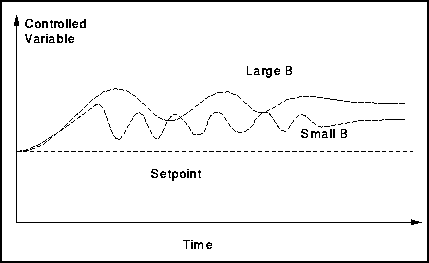As can be seen the smaller the proportional band the closer to the setpoint the controlled variable becomes but the more oscillatory the response.

The advantages of this type of controller are

• It is relativly simple and easy to design and tune
• It provides good stability
• It responds very rapidly
• Dynamically it is relatively stable

From the response curve to a step change in the input two features should be noted. These are two points which make proportional control unsatisfactory.

• Offset

For a sustained change of load, the controlled variable is not returned to the original or desired value, but attains a new equilibrium value termed control point. The difference between the control point and the desired value (set point)is referred to as offset.

The reason for this offset with proportional action is that the control action is proportional to the error. Consider the above simple level control system. For the step increase in the flow of liquid into the tank, in order to maintain the level, the valve on the outlet must be opened wider. This will only occur if there is a continuous output from the controller. The output itself can only exist if there is an error signal supplied to the controller. In order to maintain this error, the level will rise above the desired level at the new control point, hence create an offset.

• Overshoot

There is a significant time of oscillation, or in other words, overshoot. Although the period of this oscillation is moderate, this, in some cases, could be highly undesirable.

## Integral and Derivative Control

Two other common control techniques are used to eliminate the problems found when using proportional only control. These are known as integral and derivative control. Sometimes they are used individually but more often they are combined with proportional control. A more mathematical definition can be found in a later section.

• ## Integral Action

Integral action gives an output which is proportional to the time integral of the error. It is also called reset control. It is possible to use integral action itself, but this is not a common situation.

## P and I Control

Integral action is generally applied with proportional control, yielding so-called proportional and integral control (P+I). This combination is favorable in that some of the advantages of both types of control action are available.

The main advantage of P+I is that it can eliminate the offset in proportional control.

The disadvantages of P+I are that it gives rise to a higher maximum deviation, a longer response time and a longer period of oscillation than with proportional action alone. This type of control action is therefore used where the above can be tolerated and offset is undesirable.

• ## Derivative Action

This kind of action gives an output which is proportional to the derivative or the rate of change of the error. It is also known as rate control. This kind of action could not be used alone in practice. This is because its output is only related to the rate of change of the error. The error could be huge, but if it were unchanging, the controller would not give any output. Thus although it is theoretically possible, it is practically impossible.

## P and D Control

Derivative control is usually found in combination with proportional control, to form so-called P+D. By adding the derivative action, lead is added in the controller to compensate for lag around the loop, and so P+D can eliminate excessive oscillations. A disadvantage is that it can not eliminate the offset although somehow it makes it smaller.

• ## Proportional, Integral and Derivative Action

In applications, sometimes the above three action are combined together to set up the proportional plus integral plus derivative action, i.e, P+I+D.

This combined action is able to:

• eliminate the offset due to the existence of integral action
• reduce the maximum deviation and time of oscillation, which is a compromise between the advantage and disadvantage of P+I and P+D

## Examples

The aim of this module was to introduce the various types of control action. In the Case Study Section and Virtual Control Laboratory there is a level control experiment which allows the user to practice what they have learned in this section. i.e. it is possible to vary the deadzone on an on-off controller, see the effect of altering the proportional band etc.